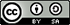# "Bridge to Mathematics"## Course Materials Include:

• Syllabus
• Instructor guide
• 2 handouts
• Solutions
• 12 example projects### Bridge to Mathematics

Mathematics Department
Northeastern University

This project-based course is designed as a 4 to 8 day (16 hour) course introducing high school students with little or no programing background to the basics of writing code with MATLAB. In this course, students will learn how to use MATLAB to analyze the basic structures of mathematics using the basic structures of programming. This course has been designed by Northeastern University for a STEM outreach program Bridge to Calculus.

#### Learning Outcomes

• Learn about mathematical topics including: plotting functions, polynomial factorization, coordinate geometry, finite sums and products, the Fibonacci sequence, probability, multivariable functions, random walks, differentiation, integration, and waves.

• Learn about programming topics including: Variables and lists, plots and their properties, functions, for loops, conditional statements, logical operands.

• Learn how to use MATLAB to:

• Create scripts and functions
• Create plots from vectors of discrete points
• Plot functions
• Use loops to compute finite sums and products
• Use loops to draw many geometric objects
• Understand the use and syntax of for loops and if-then statements
• Explore a math problem of their choice